﻿ 基以深广为例的“拍照赚钱”任务定价研究 “Photo Money” Task Pricing Research Based on Shenzhen-Guangzhou

Statistics and Application
Vol. 08  No. 04 ( 2019 ), Article ID: 31656 , 9 pages
10.12677/SA.2019.84070

“Photo Money” Task Pricing Research Based on Shenzhen-Guangzhou

Zhe Cui, Lifu Hu, Hao Li*

School of Automation, Shenyang Aerospace University, Shenyang Liaoning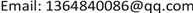Received: Jul. 19th, 2019; accepted: Aug. 1st, 2019; published: Aug. 12th, 2019ABSTRACT

This paper discusses in depth the pricing problem of new self-service crowdsourcing platform software such as “taking photos to make money”. Using the idea of mathematical modeling to study its geographical location, membership density and task pricing, the main factors affecting the pricing law are obtained. On this basis, the tasks of different heat and price are packaged together, and a set of pricing scheme is established through linear regression, cluster analysis and other methods. The final result shows that the scheme is suitable for application, the price is reasonable, and has the advantages of practicality, high accuracy, strong attraction and mutual benefit.

Keywords:Principal Component Analysis, Membership Density, Packaging Condition Model, Analytic Hierarchy Process1. 引言

2. 基于线性回归未完成任务分析

2.1. 任务空间分布分析

$E=-1.2827N+142.9765$ (1)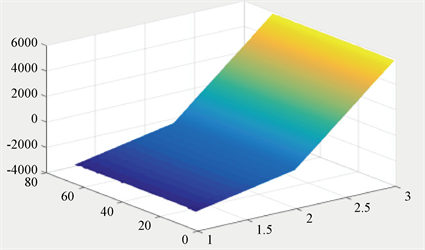(a)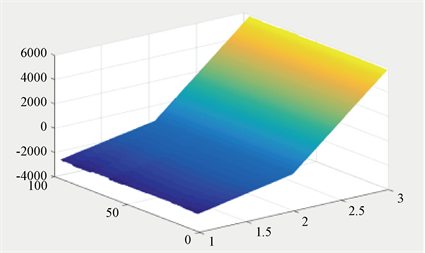(b)

Figure 1. Mission space distribution priced at 65(a) and 75(b)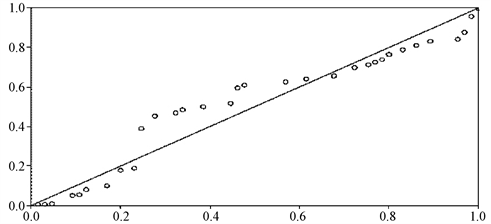Figure 2. Residual distribution

${d}_{\mathrm{max}}=0.940944,\text{ }\text{ }\text{ }\text{ }\text{ }\text{ }\text{ }\text{ }\text{ }\text{ }\text{ }\text{ }\text{ }\text{ }\text{ }\text{ }{d}_{\mathrm{min}}=0.000495$

${p}_{\mathrm{max}}=85,{p}_{\mathrm{min}}=65$

${p}_{i}={p}_{\mathrm{min}}+\frac{\left({p}_{\mathrm{max}}-{p}_{\mathrm{min}}\right)\left({d}_{i}-{d}_{\mathrm{min}}\right)}{{d}_{\mathrm{max}}-{d}_{\mathrm{min}}}$

${p}_{i}=21.27{d}_{i}+65$Figure 3. High speed image of Shenzhen and Guangzhou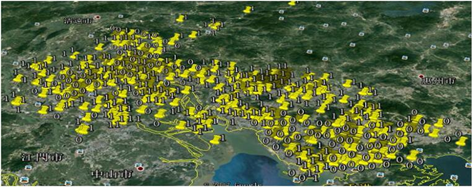Figure 4. GPS map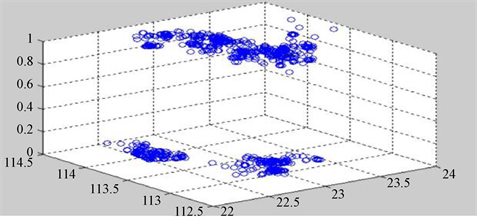Figure 5. Distribution of completion degree in different longitude and latitude

1) 无论是完成度为1还是完成度为0的任务，其定价都与完成度没有明显的关联。这也从侧面验证了我们什么对于“定价仅与地理位置有关”的猜想是正确的。

2) 完成度为1的任务点相对集中在中间区域，而完成度为0的区域相对集中在两端。因此我们猜想完成度与地理位置有一定的关联。同时也说明了原先的定价方案对于地域因素考虑的还不够全面。

3) 广州和深圳地区的任务完成度整体较低，而东莞、佛山等地的任务完成度较高。所以可能是在定价的时候忽略了不同地区的经济情况，从而导致定价不合理，部分任务完成度偏低  。

2.2. 任务价格的修缮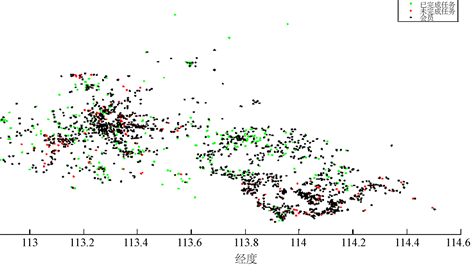Figure 6. Membership profile

2.3. 价格制定的完善

${q}_{i}={q}_{\mathrm{min}}+\frac{\left({q}_{\mathrm{max}}-{q}_{\mathrm{min}}\right)\left({t}_{i}-{t}_{\mathrm{min}}\right)}{{t}_{\mathrm{max}}-{t}_{\mathrm{min}}}$ (4)

${q}_{\mathrm{max}}=85,{q}_{\mathrm{min}}=65,{t}_{\mathrm{min}}=3.8044,{t}_{\mathrm{max}}=10863.66$

${q}_{i}=0.00184{t}_{i}+65$ (5)

${p}_{i}=21.27{d}_{i}+65$ (3)

$Q\left({p}_{i},{q}_{i}\right)=\lambda {p}_{i}+\left(1-\lambda \right){q}_{i}$

$\lambda$ 从0.1~0.9依次取值，计算出定价。

$Q=4.254d+0.001472t+65$ (6)

3. 打包任务后价格的模型

3.1. 打包标准

1) 两打包点之间的距离短。(绝对距离短)

2) 打包点之间的距离比到用户之间的距离短  。(相对距离短)

3.1.1. 打包点的确立

$E\left({D}_{w}\right)=0.02548,Z\left({D}_{w}\right)=0.01325$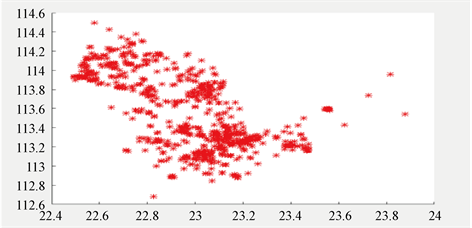${D}_{w}

$|{A}_{1}{A}_{2}|<|{A}_{1}B|,\text{\hspace{0.17em}}\text{\hspace{0.17em}}\text{\hspace{0.17em}}\text{\hspace{0.17em}}\text{\hspace{0.17em}}\text{\hspace{0.17em}}|{A}_{1}{A}_{2}|<|{A}_{2}B|$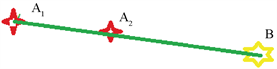Figure 8. Model diagram

3.1.2. 关于打包后任务的定价方案

4. 结语

1) 模型打包之后，任务可以更快地交付给会员们，有效提高了任务完成效率，也可以提高会员的积极性。

2) 主成分分析法是通过考虑多个因素来寻找对某一种变量的影响，具有很好的应用性，在众多相似问题中都可以用这类打包方法，比如快递跑腿，外卖配送软件、图像处理等方面。

3) 结合了SPSS，CODEBLOCKS，MAT LAB等软件，灵活地运用主成分分析法，曲线拟合等方法，使结论更加精确。

“Photo Money” Task Pricing Research Based on Shenzhen-Guangzhou[J]. 统计学与应用, 2019, 08(04): 613-621. https://doi.org/10.12677/SA.2019.84070

1. 1. 陈崇双, 唐家银, 何平. 方差分析法的线性回归建模重构[J]. 统计与决策, 2018, 34(7): 71-75.

2. 2. 徐雅静, 汪远征, 等. 主成分分析应用方法的改进[J]. 数学的实践与认识, 2006, 36(6): 68-75.

3. 3. 孙婷蔚. 统计回归模型中的主成分分析[J]. 通讯世界, 2019, 26(3): 169-170.

4. 4. 郭呈全, 陈希镇. 主成分回归的SPSS实现[J]. 统计与决策, 2011(5): 157-159.

5. 5. 金蛟. 主成分分析方法在综合评价中的应用[J]. 中国卫生统计, 2008, 25(1): 72+74.

6. 6. 贾月恬, 钟妍, 梁定康. 打包机制下的任务定价模型[J]. 中国集体经济, 2018(7): 77-78.

7. 7. 王正光. 论数学建模与数学模型[J]. 纳税, 2018(19): 237-238+242.

8. 8. 陈璇, 游小宝, 郑崇伟, 孙威, 谢胜浪. 一种新的线性回归模型及其应用示例[J]. 大气科学, 2019, 43(2): 389-400.

9. 9. 杜家菊, 陈志伟. 使用SPSS线性回归实现通径分析的方法[J]. 生物学通报, 2010, 45(2): 4-6.

10. 10. 冷建飞, 高旭, 朱嘉平. 多元线性回归统计预测模型的应用[J]. 统计与决策, 2016(7): 82-85.

11. 11. Liu, Z. (2012) Based on the Mathematical Modeling Thought Method Exploration. Advanced Materials Research, 450-451, 646-649. https://doi.org/10.4028/www.scientific.net/AMR.450-451.646

12. NOTES

*通讯作者。